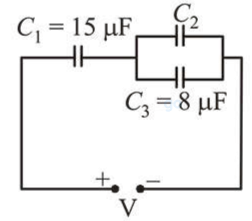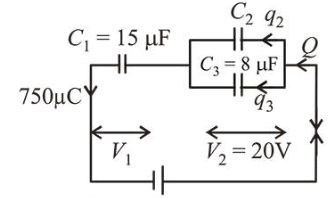# In the circuit shown in the figure,Question:

In the circuit shown in the figure, the total charge is $750 \mu \mathrm{C}$ and the voltage across capacitor $C_{2}$ is $20 \mathrm{~V}$. Then the charge on capacitor $C_{2}$ is :1. (1) $450 \mu \mathrm{C}$

2. (2) $590 \mu \mathrm{C}$

3. (3) $160 \mu \mathrm{C}$

4. (4) $650 \mu \mathrm{C}$

Correct Option: 2,

Solution:

(2) According to question,

$Q=750 \mu C=q_{2}+q_{3}$Capacitors $C_{2}$ and $C_{3}$ are in parallel hence, Voltage across $C_{2}=$ voltage across $C_{3}=20 \mathrm{~V}$ Change on capacitor $C_{3}$,

$q_{3}=C_{3} \times V_{3}=8 \times 20=160 \mu C$

$\therefore q_{2}=750 \mu C-160 \mu C=590 \mu C$